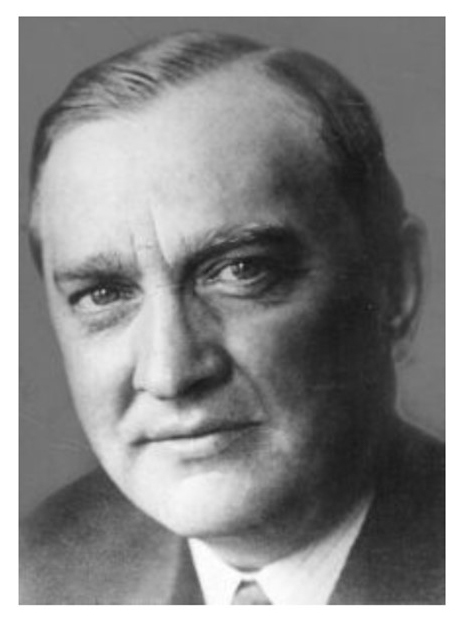# Café Mathematics II: Stefan Banach and Modern FinanceStefan Banach (1892 – 1945), Polish mathematician, who is generally considered one of the world’s most important and influential 20th-century mathematicians.

A frequent patron of the Scottish Café (see my previous post), Stefan Banach (1892 – 1945) is not a name that is normally associated with finance. He was first and foremost a mathematician, and a very famous one at that. Banach is the best known as the father of Functional Analysis, the branch of math that generalizes real analysis (finite dimensional linear algebra) to infinite dimension vector spaces. Within this broad field, Banach proved many notable theorems including a fixed-point theorem named solely after him and the Hahn-Banach Theorem, after German mathematician Hans Hahn and Banach. It is this latter theorem that provides the link between Banach and modern finance which I will discuss below, skipping most of the technical details.

We start off with a very intuitive idea that underlies all of finance theory which is called the theory of no-arbitrage pricing. This theory says that in a competitive and “frictionless” market, there shouldn’t exist risk-free opportunities to make money by trading securities such as stocks, bonds or options. When this non-arbitrage condition is satisfied, the price of a security is exactly equal to its “fair price”. Roughly speaking, you get what you pay for, no more, no less.

Theoretical economists like to think that they are applied mathematicians, and as such, would not accept even something as “obvious” as the no-arbitrage theorem without a solid proof. It turns out that the Hahn-Banach separation theorem is instrumental in proving the no-arbitrage proposition. To be a bit more precise, the mathematical concept of no-arbitrage means that you cannot start with zero wealth, trade a security, and get a payoff which is on average positive. If you could, this would constitute a riskless profit opportunity. You and other people would buy this “cheap” asset and bid up its price until the easy profit is gone and equilibrium is restored. In the language of functional analysis, equilibrium in financial markets requires that there must a set of tradable outcomes which is disjoint from the set of positive wealth. This abstract idea is best explained graphically as shown in the figure below.